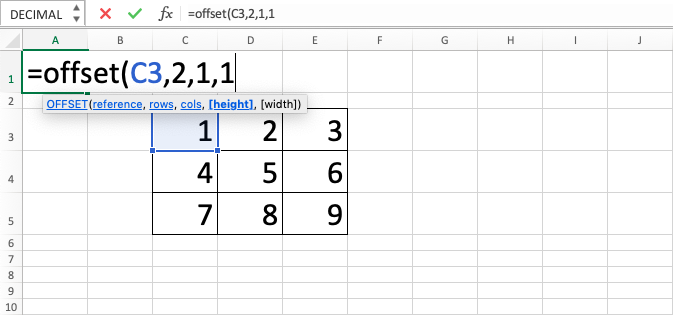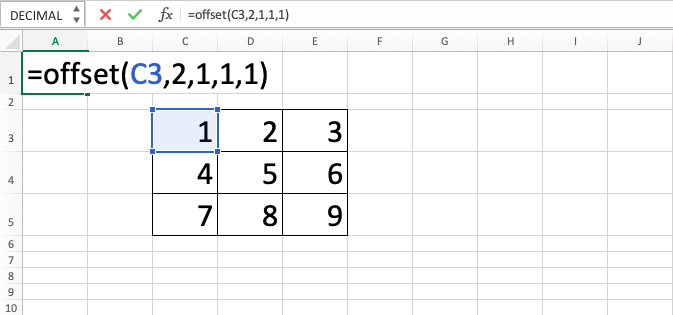OFFSET Excel Function - Compute Expert

# OFFSET Excel Function

In this tutorial, you will learn the OFFSET excel formula. OFFSET excel function can be used to get a dynamic cell coordinate reference that you can use for various purposes in the spreadsheet.

## Why do We Need to Learn OFFSET Excel Formula?

In inputting a cell coordinate reference to be used as an input in a formula or utilized in other functions in a spreadsheet, sometimes we want the reference to be dynamic. This is so the formula or function result that we want to use can anticipate the change from the shift of our data reference location in the spreadsheet.

As an example, imagine we want to show the additional process result from some of the numbers in a group that is saved in the spreadsheet. The process is done using the SUM formula. We might want the result of the SUM that is placed in a cell to be dynamic from the reference input side so it can be adapted with the additional process result that we want to show to other people and also to make the interface of the spreadsheet simpler. By inputting the shift of the cell row and column as desired, we want the SUM coordinate input reference to be changed directly without having to write the formula all over again.

Or maybe we want to make a dropdown with a dynamic and changing options depending on the data that we input in the spreadsheet. When the relevant data is added, deleted, or modified, we want the dropdown choices to also be changed automatically corresponding with the revision of our data.

If the dynamic cell coordinate that we want is needed in a lot of formulas or functions, then it will be very troublesome if we want to do the change process manually in each formula or function where they are inputted. To help with this problem, you can use the OFFSET excel function.

OFFSET excel formula can help to make a cell coordinate reference to become flexible as the needs in the two cases above. OFFSET excel function is very useful to be learned and used if you need a dynamic reference and result in the data processing on the spreadsheet.

## What is OFFSET Excel Function?

OFFSET excel function is a function with the usage objective to make your cell coordinate reference becomes dynamic related to the change of data that is saved in it. OFFSET excel formula is often combined with another formula or function to help add the dynamic attribute for the formula or function input reference whenever needed.

Generally, this is the OFFSET excel formula inputs’ explanation:

=OFFSET(reference, rows, cols, [height], [width])

Notes:
• reference = the initial cell or range coordinate to be given the dynamic attribute
• rows = the amount of position shift to the bottom as desired from the initial coordinate
• cols = the amount of position shift to the right as desired from the initial coordinate
• [height] = optional. The number of cells from the most top-left of the initial one to the bottom that you want to involve in the reference result later
• [width] = optional. The number of cells from the most top-left of the initial one to the right that you want to involve in the reference result later

## How to Use OFFSET Excel Formula?

The following will explain the writing of the OFFSET excel function. OFFSET excel formula needs three required inputs which are the initial cell or range coordinate before the dynamic process is done, the amount of shift from the initial position to the bottom, and the amount of shift to the right. Besides that, there are two optional inputs for OFFSET excel formula which are the number of cells from the most top-left of the initial one to the bottom and to the right positions that you want to be involved as the result of the dynamic process of your cell or range coordinate.

## Using OFFSET Excel Function

1. Type equal sign ( = ) in the cell where you want to put the process result in2. Type OFFSET (can be with large and small letters) and open bracket sign after =3. Input the initial cell or range coordinate and type a comma sign ( , )4. Input the amount of shift to the bottom or cell coordinate where the number is in then type a comma sign ( , )5. Input the amount of shift to the right or cell coordinate where the number is in6. Optional: Type a comma sign then input the number of cells from the most top-left of the initial one to the bottom that you want to involve in the result later / the coordinate where the number is. If the input on this part is not given, then it will follow the number from the initial cell or range that is inputted previously7. Optional: Type a comma sign then input the number of cells from the most top-left of the initial one to the right that you want to involve in the result later / the coordinate where the number is. If the input on this part is not given, then it will follow the number from the initial cell or range that is inputted previously8. Type close bracket sign9. Type Enter
10. The process of OFFSET excel function is done!## Exercise

After you have done the learning of how to write the OFFSET excel formula by following the steps above, now is the time you work on the exercise about OFFSET excel function to sharpen your understanding!

Link for the exercise file of OFFSET excel formula:

### Questions

1. Get the number of E2 from the initial position of A1!
2. Sum all the numbers from C4 to E5 using SUM with the initial position of A2!
3. Get the average of all the numbers from A1 to C3 using AVERAGE with the initial position of C3!

• If you want to do shift to the top or the left from the initial coordinate in the OFFSET excel formula, then you can input a minus number in the shift amount part of the input with the amount of shift that you want
• If you do the shift of the dynamic reference to the outside of the spreadsheet scope (e.g. shift A1 to the left or to the top where there is not any cell left in the spreadsheet), then OFFSET excel function will result in #REF error

Get updated excel info from Compute Expert by registering your email. It's free!